## Example Questions

← Previous 1

### Example Question #33 : Percentage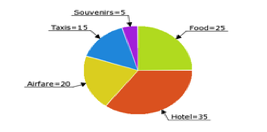David's trip expenses are pictured in the above pie chart (numbers = % of his total expenses). If he spent $75 on taxis, how much did he spend on hotel and souvenirs combined? Possible Answers:$40

$175$225

$250$200

$200 Explanation: David spent$75 on taxis, which were 15% of his total expenses on the trip. He therefore spent 75(100/15) = $500 on the trip altogether. The hotel and souvenirs make up 35% + 5% = 40% of his total expenses. 40% of 500 is$200.

### Example Question #34 : Percentage

30% of what number is 20?

Solve to the nearest hundredth.

150%

1.5

66.67

0.67

66.67

Explanation:

This is a very basic form percentage question. This can be rewritten:

0.3 * x = 20

(Remember, the word "of" in a word problem indicates multiplication, while the word "is" indicates an equals sign).

Solve for x: x = 20 / 0.3 = 66.67

A toy is on sale for 43% off. Its sale price is $21.37. What is the full price? Possible Answers: None of the available answers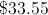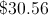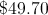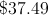Correct answer:Explanation: An algebraic expression for this item is: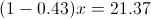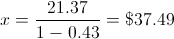### Example Question #35 : Percentage Twenty-six students planned to contribute an equal amount to purchase a gift for their teacher. After 18 students had paid, they had collected$76.50. What is the total price of the gift?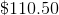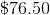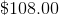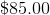Explanation:

If $76.50 had been collected after 18 students had paid, we can determine how much each student contributed:$76.50/18 = $4.25 per student Now we can multiply this by the total number of students (26) to get the full price of the gift: 26 x$4.25 = \$110.50

### Example Question #1 : Whole And Part

If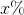ofis, then what isof?

10

100

20

200

50

50

Explanation:

The first part of the problem tells us that x% of 20 is 50. We can model x% as x/100 or 0.01x. To find x% of 20, we can multiply 0.01x and 20. In other words, we can write the following equation:

(0.01x)(20) = 50

Divide both sides by 20.

0.01x = 2.5

Divide both sides by 0.01.

x = 250.

The question then asks us to find 20% of x. We can represent 20% as 0.2, and we know that x is 250. Therefore,

20% of 250 = 0.2(250) = 50.

### Example Question #1 : How To Find The Whole From The Part With Percentage

Ifof a number is, what isof the number?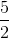Explanation:

Let x be the number in question.

Then 2/3 * x = 18.

x = 18 * 3/2 = 27

Now find 1/9 of 27:

1/9 * 27 = 3

### Example Question #1 : How To Find The Whole From The Part With Percentage

17 is 85% of what number?Explanation:

In this case, 17 is part of a whole x. We are also given that 17 is 85% of x.

With this in mind, we can set up the following proportion.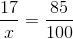To solve for x, cross multiply.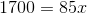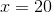### Example Question #2 : How To Find The Whole From The Part With Percentage

On the ride home from school, Jim notices thatpecent of the cars he passes are yellow. Jim seesyellow cars. How many cars does Jim see that aren't yellow?Explanation:

Let x represent the total number of cars Jim sees on the way home. If Jim sees 7 yellow cars and notices that 35% of all the cars he sees are yellow, then we can express this as

7/x = 35%, or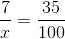Using cross multiplication, we see that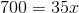Therefore, Jim sees 20 total cars on the way home. The question wants to know how many cars are NOT yellow. We can find that by subtracting the total number of cars from the number of yellow cars: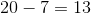### Example Question #3 : How To Find The Whole From The Part With Percentageis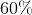of what number?None of the given answers are correct.Explanation:

To solve this problem, we can set up a proportion and solve for our missing value using cross multiplication.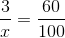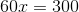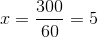### Example Question #4 : How To Find The Whole From The Part With Percentage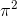is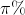of what number?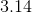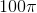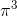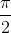Explanation:

Don't let the pi throw you off on this question. We can set up a proportion to solve for the whole that we are looking for.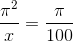(Remember that any percentage can also be written as a fraction.)

Now we can solve for our unknown by using cross multiplication.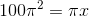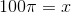Therefore,isof← Previous 1